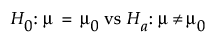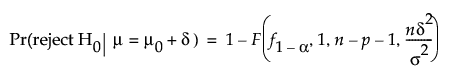Design of Experiments Guide > Prospective Sample Size and Power > One Sample Mean Calculator > Statistical Details for the One Sample Mean Calculator
Publication date: 11/10/2021

## Statistical Details for the One Sample Mean Calculator

The one sample mean calculations are based on the traditional full versus reduced F test for the following hypothesis test:JMP calculates power as follows:where:

α is the significance level.

n is the sample size.

p is the number of extra parameters.

δ is the difference to detect.

f1-α is the (1 - α)th quantile of the F(1, n- p -1) distribution.

F(x, df1, df2, nc) is the cumulative distribution function of the non-central F distribution with degrees of freedom df1 and df2 and non-centrality parameter nc evaluated at x.

Because analytical solutions for δ and n do not exist, numerical solutions are used to solve for them.